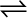7.28 Dihydrogen gas is obtained from natural gas by partial oxidation with steam as per the following endothermic reaction:

CH4 (g) + H2O (g)CO (g) + 3H2 (g)

(a) Write as an expression for Kp for the above reaction.

(b) How will the values of Kp and composition of equilibrium mixture be affected by

(i) increasing the pressure

(ii) increasing the temperature

(iii) using a catalyst?

(a) For the given reaction,

${\mathrm{K}}_{\mathrm{p}}=\frac{{\mathrm{p}}_{\mathrm{co}}×{{\mathrm{p}}^{3}}_{{\mathrm{H}}_{2}}}{{\mathrm{p}}_{{\mathrm{CH}}_{4}×{\mathrm{p}}_{{\mathrm{H}}_{2}\mathrm{O}}}}$

(b) (i) According to Le Chatelier’s principle, the equilibrium will shift in the backward direction.

(ii) According to Le Chatelier’s principle, as the reaction is endothermic, the equilibrium will shift in the forward direction.

(iii) The equilibrium of the reaction is not affected by the presence of a catalyst. A catalyst only increases the rate of a reaction. Thus, equilibrium will be attained quickly.